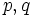# Right residual operator for composition

## Contents

BEWARE! This term is nonstandard and is being used locally within the wiki. [SHOW MORE]

This is a binary subgroup property operator, viz an operator that takes as input two subgroup properties, and outputs one subgroup property

## Definition

### Property-theoretic statement

Suppose$p,q$ are two subgroup properties. The right residual of$p$ by$q$ is the unique subgroup property$r$ such that:$q * s \le p \iff s \le r$.

Here,$*$ denotes the composition operator.

### Statement with symbols

Suppose$p,q$ are two subgroup properties. The right residual of$p$ by$q$ is defined as the subgroup property$r$ as follows:$H$ has property$r$ in$G$ if whenever$K$ is a subgroup of$H$ with property$q$ in$H$,$K$ has property$p$ in$G$.

## Facts

### Relation with right transiter

Further information: Right transiter

The right transiter of a subgroup property$p$ is defined as the right residual of$p$ by itself.